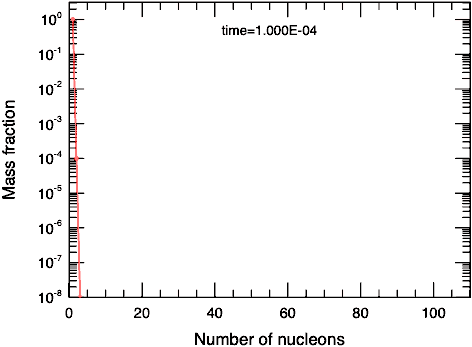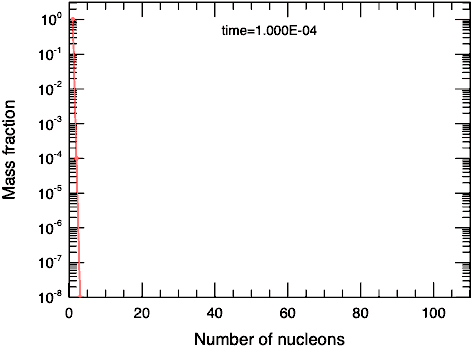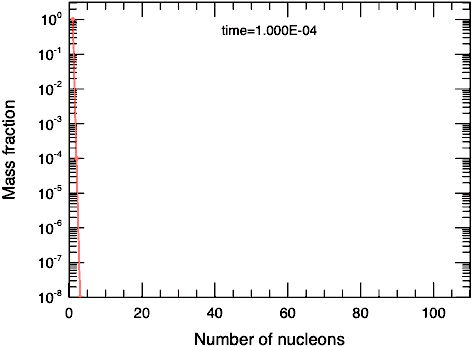Cococubed.com Slow Neutron Captures

Home

Astronomy research
Software instruments
Stellar equation of states
EOS with ionization
EOS for supernovae
Chemical potentials
Stellar atmospheres

Voigt Function
Jeans escape
Polytropic stars
Cold white dwarfs

Cold neutron stars
Stellar opacities
Neutrino energy loss rates
Ephemeris routines
Fermi-Dirac functions

Polyhedra volume
Plane - cube intersection
Coating an ellipsoid

Nuclear reaction networks
Nuclear statistical equilibrium
Laminar deflagrations
CJ detonations
ZND detonations

Fitting to conic sections
Unusual linear algebra
Derivatives on uneven grids

Supernova light curves
Exact Riemann solutions
1D PPM hydrodynamics
Hydrodynamic test cases
Galactic chemical evolution

Universal two-body problem
Circular and elliptical 3 body
The pendulum
Phyllotaxis

MESA
MESA-Web
FLASH

Zingale's software
Brown's dStar
GR1D code
Herwig's NuGRID
Meyer's NetNuc
Presentations
Illustrations
Public Outreach
Education materials
2022 ASU Solar Systems Astronomy
2022 ASU Energy in Everyday Life

AAS Journals
2022 Earendel, A Highly Magnified Star
2022 TV Columbae, Micronova
2022 White Dwarfs and 12C(α,γ)16O
2022 MESA in Don't Look Up
2022 MESA Marketplace
2022 MESA Summer School
2022 MESA Classroom
2021 Bill Paxton, Tinsley Prize

Contact: F.X.Timmes
my one page vitae,
full vitae,
research statement, and
teaching statement.

The tool minis.tbz evolves an educational version of an s-process reaction network. One hundred isotopes are evolved until a chosen ending time. The initial abundance of the first isotope, notionally 56Fe, is taken equal to one. Guidance on what this tool does seems prudent. Let $R$ be the reaction rate for (n,g) reactions. In general $R$ is temperature, density, and composition dependent - but not here. The ordinary differential equations describing the change in the abundances $y$ of the $m$ isotopes are: \begin{equation} \frac{{\rm d}y_{1}}{{\rm d}t} = -y_{1} - R_{1} \hskip 0.5in \frac{{\rm d}y_{i}}{{\rm d}t} = y_{i-1} \ R_{i-1} - y_{i} \ R_{i} \ , \ i=1,2\ldots,m-1 \hskip 0.5in \frac{{\rm d}y_{m}}{{\rm d}t} = y_{m-1} \ R_{m-1} \label{eq1} \tag{1} \end{equation} For the implicit first-order accurate Euler method, each abundance is updated over a timestep h as $y_{i}^{{\rm new}} = y_{i} + \Delta y_{i}$. The change in the abundances over a time step $\Delta y_{i}$ is obtained from solving the system of linear equations $({\bf I}/h - \tilde{{\bf J}}) \cdot \Delta {\bf y} = \dot{\bf y}$, which is simply the familar $\tilde{{\bf A}} \cdot {\bf x} = {\bf b}$. With only (n,g) reactions, Jacobian matrix $\tilde{{\bf J}}$ has the simple form \begin{equation} \left[\begin{array}{rrrrrr} -R_{1} & & & & & \\ R_{1} & -R_{2} & & & & \\ & R_{2} & -R_{3} & & & \\ & & & \ldots & & \\ & & & & R_{m-1} & 0 \\ \end{array}\right] \label{eq2} \tag{2} \end{equation} This system of linear equations can be easily solved by hand: \begin{equation} \Delta y_1 = \frac{-y_1 R_1}{1/h + R_1} \hskip 0.5in \Delta y_i = \frac { y_{i-1} R_{i-1} - y_i R_i } {1/h + R_i} \ , \ i=1,2\ldots,m-1 \hskip 0.5in \Delta y_m = \frac{-y_{m-1} R_{m-1}}{1/h} \label{eq3} \tag{3} \end{equation} Thus the succint evolution loop implemented in minis.tbz. Here are some results:All exposures equal to oneA middle exposure at 0.1A middle exposure at 10.0

Please cite the relevant references if you publish a piece of work that use these codes, pieces of these codes, or modified versions of them. Offer co-authorship of the publication if appropriate. At best, you'll love these programs so much that you'll send great wads of cash to me.